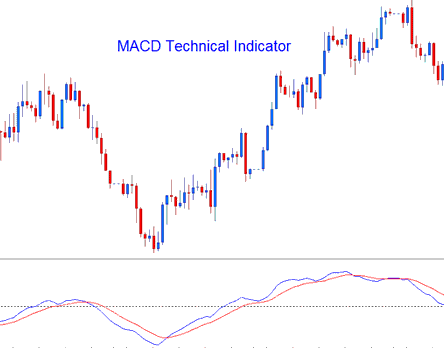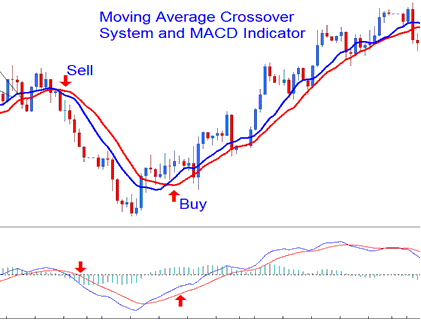# MACD - Moving Average Convergence/Divergence Forex Technical Analysis and MACD Forex Trading Signals

Developed by Gerald Appel,

The Moving Average Convergence/Divergence is one of the simplest, reliable, and most commonly used indicators.

It is a momentum oscillator and also a trend-following indicator.

### Construction

The construction of this indicator calculates the difference between two moving averages and then plots that as the "Fast" line; the a second "Signal" line is then calculated from the resulting "Fast" line and then plotted on the same panel window as the "Fast" line.

• "Fast" line – Blue Line

• "Signal" line– Red Line

The "standard" MACD values for the "Fast" line is a 12-period exponential moving average and a 26-period exponential moving average and a 9-period exponential moving applied to the fast line, this plots the "Signal" line.

• Fast line= difference between 12 and 26 exponential moving averages

• Signal line= moving average of this difference of 9-periods## Forex Technical Analysis and Generating Forex Trading Signals

The MACD is commonly used as a trend-following indicator and works most effectively when analyzing trending market movements. The three common methods of using MACD to generate signals are:

### Crossovers Signals:

Fast line/Signal line Crossover:

• A buy signal is generated when the Fast line crosses above the Signal line

• A sell signal is generated when the Fast line crosses below the Signal line.

However, in a strong trending market this signal gives a lot of whipsaws, the best crossover to use would thus be the Zero Line Crossover Signal that is less prone to whipsaws.

Zero Line Crossover Signals:

• When the Fast line crosses above zero center line a buy signal is generated.

• when the Fast line crosses below zero center line a sell signal is generated.Looking for divergences between the MACD and price can prove to be very effective in identifying potential reversal and/or trend continuation points in price movement. There two types of divergences:

1. Classic Divergence Signals

2. Hidden Divergence Signals

### Overbought/Oversold Conditions:

MACD is also used to identify potential overbought/oversold conditions in price action movements.

These levels are generated if the shorter MACD Lines separate dramatically from the median, this is an indication that price action is over-extending & it will soon return to more realistic levels.

### MACD and Moving Average Crossover System

This indicator can be combined with others to form a Forex trading system. A good combination with the Moving Average crossover system. A trading signal is generated when both give a signal in the same direction.Technical Analysis